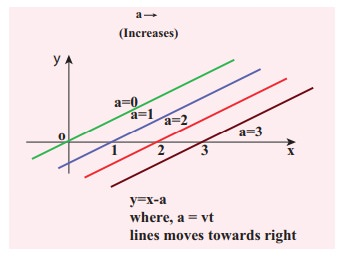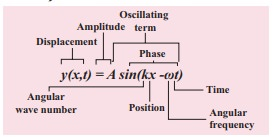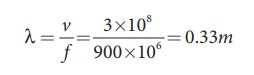Home | | Physics 11th std | Solved Example Problems for Progressive Waves (or) Travelling Waves

# Solved Example Problems for Progressive Waves (or) Travelling Waves

Physics : Waves - Solved Example Problems for Progressive Waves (or) Travelling Waves

### EXAMPLE 11.11

Sketch y = x −a for different values of a.

### SolutionThis implies, when increasing the value of a, the line shifts towards right side. For = vt, y = x  vt satisfies the differential equation. Though this function satisfies the differential equation, it is not finite for all values of x and t. Hence, it does not represent a wave.

### EXAMPLE 11.12

How does the wave y = sin(x − a) for a = 0, a = π/4, a = π/2, a = 3π/2, a = π look like?. Sketch this wave.

### SolutionFrom the above picture we observe that y = sin (x−a) for a = 0, a = π/4, a = π/2, a = 3π/2 and a = π, the function y = sin (x−a) shifts towards right. Further, we can take a = vt and v = π/4 ,  and sketching for different times t = 0st = 1 st = 2s etc., we once again observe that y = sin(xvt) moves towards the right. Hence, y = sin(xvt) is a travelling (or progressive) wave moving towards the right. If y = sin(x+vt) then the travelling (or progressive) wave moves towards the left. Thus, any arbitrary function of type = f(xvt) characterising the wave must move towards right and similarly, any arbitrary function of type y = f(x+vt) characterizing the wave must move towards left.

### EXAMPLE 11.13

Check the dimensional of the wave y = sin(xvt). If it is dimensionally wrong, write the above equation in the correct form.

### Solution

Dimensionally it is not correct. we know that y = sin(xvt) must be a dimensionless quantity but xvt has dimension. The correct equation is y = sin (k xωt), where and ω have the dimensions of inverse of length and inverse of time respectively. The sine functions and cosine functions are periodic functions with period 2π. Therefore, the correct expression is y = sin ([2π/λ]x, [2π/T]t) where λ and T are wavelength and time period, respectively.

In general, y(x,t)=A sin(k xωt).### EXAMPLE 11.14

The wavelength of two sine waves are λ1 = 1m and λ2 = 6m. Calculate the corresponding wave numbers.

### Solution### EXAMPLE 11.15

A mobile phone tower transmits a wave signal of frequency 900MHz. Calculate the length of the waves transmitted from the mobile phone tower.

### Solution

Frequency, f = 900 MHz = 900 ×106 Hz

The speed of wave is c = 3 × 108m s−1Tags : Physics , 11th Physics : UNIT 11 : Waves
Study Material, Lecturing Notes, Assignment, Reference, Wiki description explanation, brief detail
11th Physics : UNIT 11 : Waves : Solved Example Problems for Progressive Waves (or) Travelling Waves | Physics